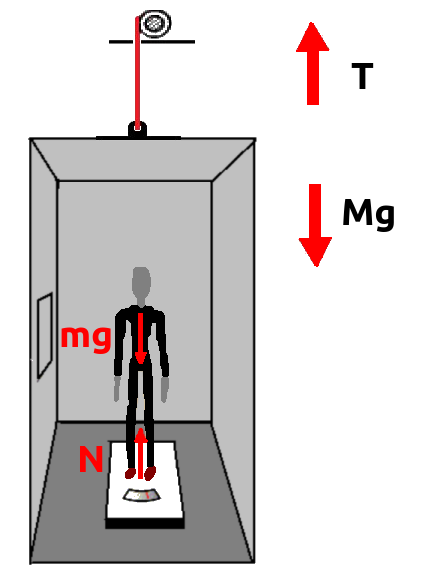Inside an elevator you feel heavier, lighter, or normal depending on the motion of the elevator.  In fact what you feel as your weight is your apparent weight, that is not always the same as your actual weight (or simply your weight). In this article you will find the definition of apparent weight and actual weight. Besides, then the motion of elevator and the change of your apparent weight inside it will be discussed.## How Does Your Weight Change in an Elevator?

1

### Apparent weight and actual weight

The actual weight (or weight) of an object on earth is the gravitational force exerted by the earth on the object. That is given by:

W=mg

"M" is the mass of the object and "g" is the gravitational acceleration.

But the weight that you feel is apparent weight, that is the normal force (or the support force) exerted on you by the surface that is in contact with you. This force is the reaction force of the force you exert upon the surface, and it is not always the same as gravitational force acting on you. For instance when you are in free fall, you do not exert any force on the surface that is in contact with you, so the normal force acting on you is zero, and you feel weightless.

2

### Equations of motion of elevator and a passenger inside it

Forces acting on an elevator (and everything inside it) are gravitational force "Mg", and an upward force that is the force of tension "T", in the cable of elevator, as shown in the picture. So if you assume that upward direction is positive and downward direction is negative, according to the Newton's second law, the equation of motion of elevator can be written as:

T-Mg=Ma

"T-Mg" is the net force acting on elevator, and "M" is the total mass of elevator. "A" is the acceleration rate of elevator. When the net force is positive, "a" is upward and vice versa.

On the other hand, the forces acting on a passenger with mass "m" who is standing on a scale, are gravitational force, "mg", and an upward normal force "N", exerted by the surface of scale. So the equation of motion of passenger is:

N-mg=ma

"N" is the apparent weight of passenger (the reading on the scale), and "mg" is actual weight of passenger.

So apparent weight of passenger is given by:

Wapp. =N= m(g+a)

And the apparent weight of elevator is equal to "T".

When elevator is standing still or moving with constant speed, "a" is zero and the apparent weight of passenger is equal to his/her actual weight.

3

### Upward motion of elevator

Upward motion of elevator contains three stages:

1. Initial stage with upward acceleration:
When the elevator is starting to move upward (from a lower floor to an upper floor), the initial net force acting on it must be upward (provided by increased tension on the cable, T>Mg). This initial net force provides an upward acceleration initially. So initial value of "a" is positive and the passenger feels heavier according to the Wapp.=m(g+a), and if he/she is carrying a bag it feels heavier too.

2. Middle stage with zero acceleration:
After starting the upward motion, the net force acting on elevator is zero (T=Mg) and elevator continues its upward motion with a constant speed. So at this stage, "a" is zero and apparent weight of passenger is the same as his/her actual weight (he/she feels normal).

3.  Final stage with downward acceleration:
When the elevator is going to stop at the upper floor, its speed decreases by a downward acceleration (provided by decreased tension on the cable, T<Mg); so at this stage "a" is negative and the apparent weight of passenger is smaller than his/her actual weight (the passenger feels lighter, and if he/she is carrying a bag, it feels lighter too).

4

### Downward motion of elevator

Downward motion of elevator also contains three stages:

1. Initial stage with downward acceleration:
When the elevator is starting to move downward (from an upper floor to a lower floor), the initial net force acting on it must be downward (provided by decreased tension on the cable, T<Mg). This initial net force provides a downward acceleration initially. So initial value of "a" is negative and the passenger feels lighter according to the Wapp.=m(g+a), and if he/she is carrying a bag it feels lighter too.

2. Middle stage with zero acceleration:
After starting the downward motion, the net force acting on elevator is zero (T=Mg) and elevator continues its downward motion with a constant speed. So at this stage, "a" is zero and apparent weight of passenger is the same as his/her actual weight (he/she feels normal).

3.  Final stage with upward acceleration:
When the elevator is going to stop at the lower floor, its speed decreases by a upward acceleration (provided by increased tension on the cable, T>Mg); so "a" is positive and the apparent weight of passenger is larger than his/her actual weight (the passenger feels heavier, and if he/she is carrying a bag it feels heavier too).

Tips
• You can find problem solving lectures about this topic and a comprehensive discussion in different topics of Classical Mechanics in college entrance level at: https://www.udemy.com/physics-12-part-1-classical-mechanics/